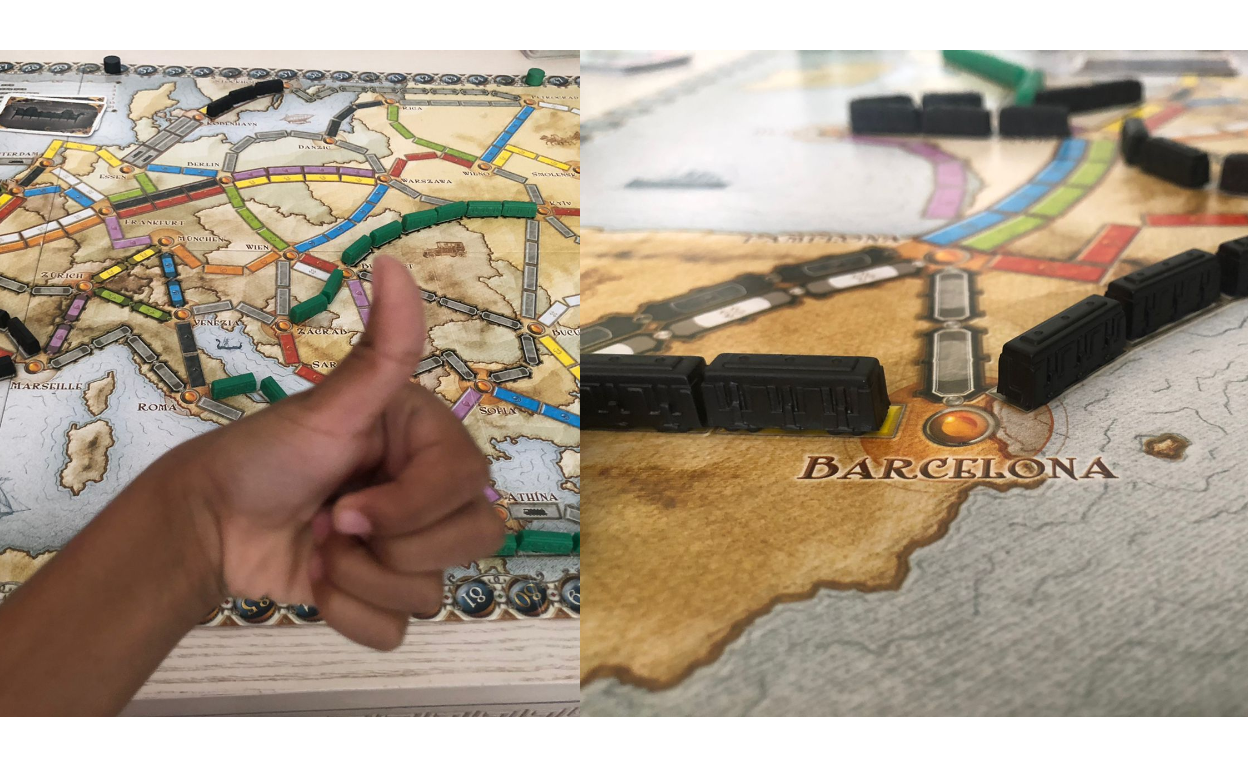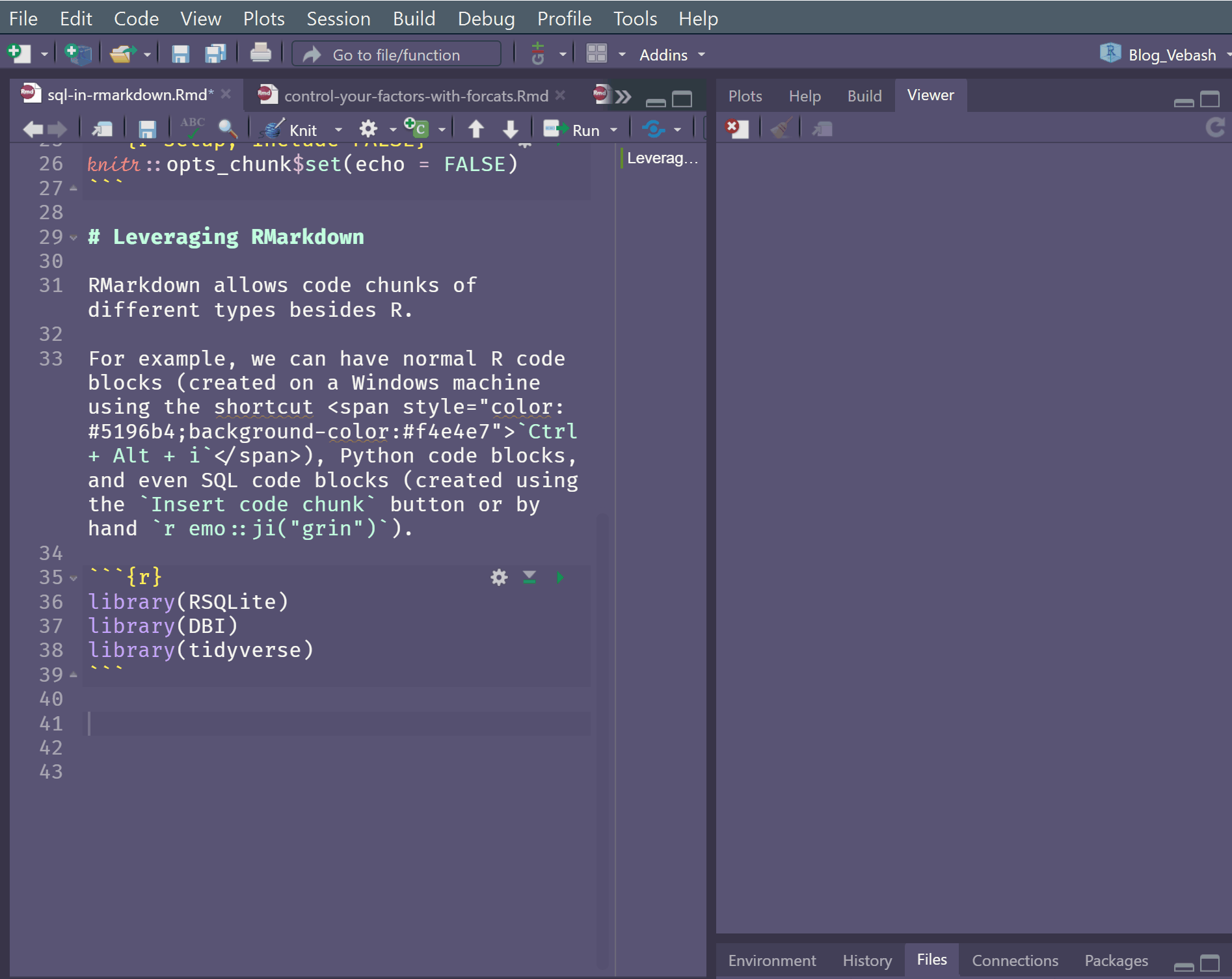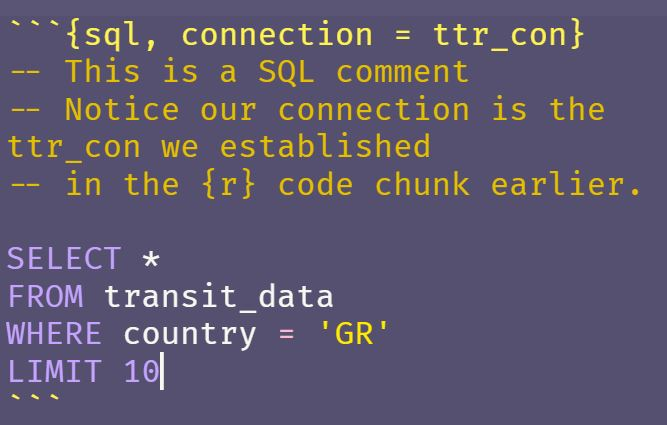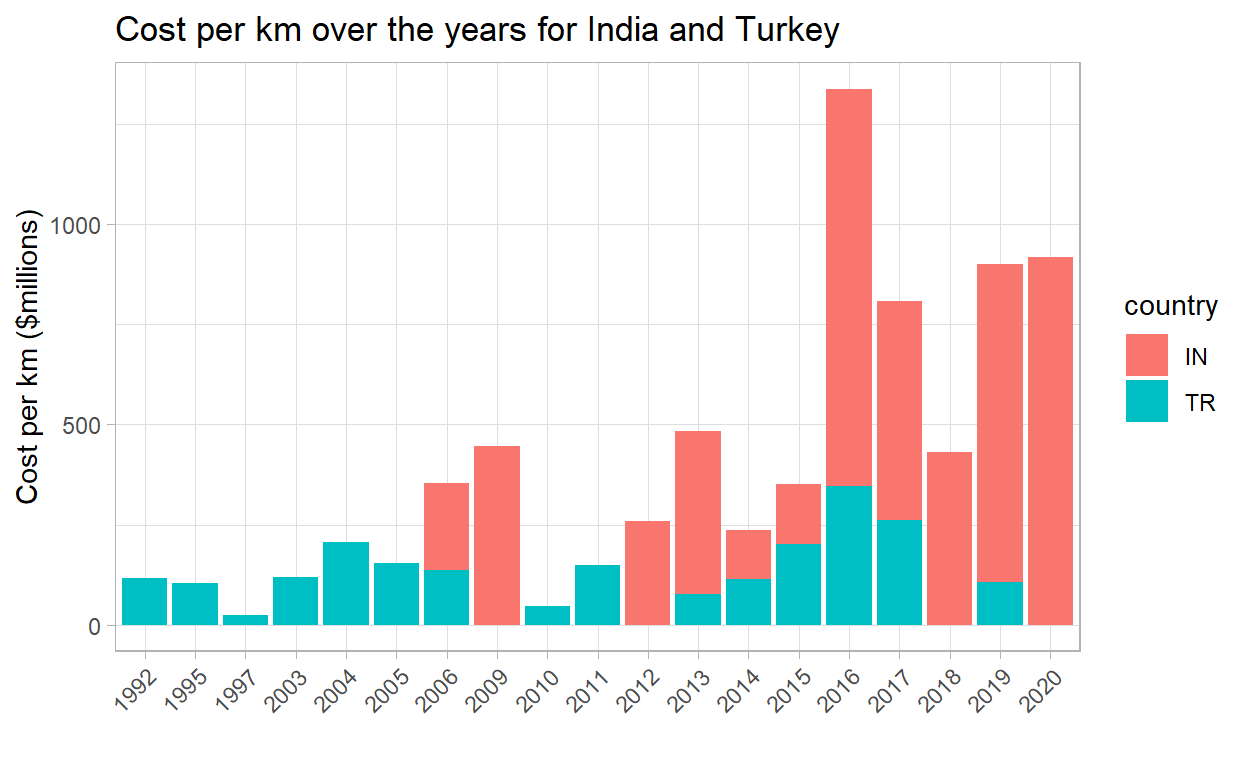# sql-in-rmarkdown

We’re going to mix {sql} and {r} code chunks in a .Rmd file to leverage our knowledge in both languages.

2021-03-25# Leveraging RMarkdown

RMarkdown (Rmd) allows code chunks of different language types besides R.

For example, we can have normal R code blocks (created on a Windows machine using the shortcut `Ctrl + Alt + i`), Python code blocks, and even SQL code blocks (created using the `Insert code chunk` button, or by hand 😄).In this post we’re going to write `SQL` syntax and intertwine that with R code to enable you to use both, if you are familiar with both.

We will also look at the `{tidyquery}` package which allows you to learn {dplyr} if you’re more familiar with SQL, but keen to grasp more {dplyr}.

## Prerequisites

The blog post assumes that you have at least a beginner understanding of:

1. RMarkdown
2. SQL
3. Tidyverse
4. Connecting to a DB from RStudio (see Part I, Part II or Part III for more info).

## Dataset

In my home, my 6 year old is currently obsessed with Ticket to Ride Europe, so I was excited when I saw the TidyTuesday Transit Costs Project data 🚂.

### Glimpse into the Data

To work with a Database in R we need a few packages. There are a few blog posts on this site that contains more info on how this is achieved, for example, Using the Tidyverse with Databases - Part I.

``````library(DBI) # main DB interface
library(RSQLite) # To connect to SQLite DB
library(tidyverse) # for dplyr, readr etc.
library(dbplyr) # dplyr backend for DBs
``````
``````# read in the TidyTuesday dataset
'rfordatascience/tidytuesday/master/data/',
'2021/2021-01-05/transit_cost.csv'))
transit_cost %>%
DT::datatable(list(scrollX = TRUE,
pageLength = 5))
``````

If we look at the data we see that `Country` is the 2-letter code CZ, DK, CH etc. We can use the `{countrycode}` package to find the actual country name.

Let’s take a look at a few of the variables included in the `countrycode::codelist` dataframe.

``````library(countrycode)
codelist %>%
select(country.name.en,
country.name.en.regex,
iso.name.en,
currency,
iso2c, iso3c)
``````
``````# A tibble: 288 x 6
country.name.en   country.name.en~ iso.name.en currency iso2c iso3c
<chr>             <chr>            <chr>       <chr>    <chr> <chr>
1 Afghanistan       afghan           Afghanistan Afghani  AF    AFG
2 Åland Islands     ^[å|a]land       Åland Isla~ Euro     AX    ALA
3 Albania           albania          Albania     Lek      AL    ALB
4 Algeria           algeria          Algeria     Algeria~ DZ    DZA
5 American Samoa    ^(?=.*americ).*~ American S~ US Doll~ AS    ASM
6 Andorra           andorra          Andorra     Euro     AD    AND
7 Angola            angola           Angola      Kwanza   AO    AGO
8 Anguilla          anguill?a        Anguilla    East Ca~ AI    AIA
9 Antarctica        antarctica       Antarctica  <NA>     AQ    ATA
10 Antigua & Barbuda antigua          Antigua an~ East Ca~ AG    ATG
# ... with 278 more rows``````

If you try the countrycode::countrycode() function you will get a warning for `UK` in the `transit_cost` dataset. This is because the United Kingdom is coded as GB not UK. So we need to amend our dataset first before we try and use the function.

``````transit_cost %>%
# rename UK as GB to enable
# the join later
mutate(country = if_else(country == "UK",
"GB",
country)) %>%
mutate(country_name = countrycode(country, origin = 'iso2c',
destination = "country.name")) %>%
count(country, country_name, sort = TRUE) %>%
DT::datatable(list(scrollX = TRUE,
pageLength = 8))
``````

Now we can see which country is attached to which `iso2` code, DK = Denmark, CN = China etc.

The `codelist` dataframe contains the raw data for countries as we saw previously.

Show code
``````codelist %>%
``````
``````# A tibble: 4 x 743
ar5      cctld continent country.name.de country.name.de.regex
<chr>    <chr> <chr>     <chr>           <chr>
1 ASIA     .af   Asia      Afghanistan     afghan
2 OECD1990 .ax   Europe    Aland Islands   åland
3 EIT      .al   Europe    Albanien        albanien
4 MAF      .dz   Africa    Algerien        algerien
# ... with 738 more variables: country.name.en <chr>,
#   country.name.en.regex <chr>, cow.name <chr>, cowc <chr>,
#   cown <dbl>, currency <chr>, dhs <chr>, ecb <chr>, eu28 <chr>,
#   eurocontrol_pru <chr>, eurocontrol_statfor <chr>, eurostat <chr>,
#   fao <dbl>, fips <chr>, gaul <dbl>, genc2c <chr>, genc3c <chr>,
#   genc3n <chr>, gwc <chr>, gwn <dbl>, icao.region <chr>, imf <dbl>,
#   ioc <chr>, iso.name.en <chr>, iso.name.fr <chr>, iso2c <chr>, ...``````

## Write data to a SQLite DB

We’re going to write the `transit_cost` and `codelist` dataframes into tables in a SQLite DB for our demo.

``````# Make a connection to a non-existant
# DB - here the DB `ticket_to_ride` does
# not yet exist.
# This step creates it.
con <- DBI::dbConnect(RSQLite::SQLite(),
dbname = "ticket_to_ride.sqlite")
``````
``````# Amend UK to GB for the join of country names later
transit_cost <- transit_cost %>%
mutate(country = if_else(country == "UK",
"GB",
country))

# rename to an easy to understand SQL table name
country_codes <- codelist

# Write dataframes into DB tables
dbWriteTable(con,
"transit_cost", # table will be named transit_cost
transit_cost, # df being written
overwrite = TRUE)
dbWriteTable(con,
"country_codes", # table will be named country_codes
country_codes, # df being written
overwrite = TRUE)

# close the connection
dbDisconnect(con)
``````

I closed the connection here since the tasks of writing data into a Database, and reading from it, are most likely separate.

In this blog post these functions run into each other, and we don’t strictly need to do this `dbDisconnect(con)` here.

## Connect to the Database

Now that we have a SQLite DB, we can connect to it and query the data we have stored there.

``````# Make a connection
ttr_con <- dbConnect(RSQLite::SQLite(), "ticket_to_ride.sqlite")
# List tables in DB
dbListTables(ttr_con)
``````
`` "country_codes" "transit_cost" ``

Ah nice, we see our two tables. Let’s have a closer look.

``````# Get a glimpse of the data

# Using our connection reach into the DB
# and show us some of the data for GR

# Equivalent to:
#   SELECT * FROM transit_cost
#   WHERE country = 'GR'
tbl(ttr_con, "transit_cost") %>%
filter(country == "GR")
``````
``````# Source:   lazy query [?? x 20]
# Database: sqlite 3.36.0 [C:\Users\Vebashini\OneDrive -
#   Rain\Documents\Main-Documents\Personal\Blog_Vebash\_posts\2021-03-27-sql-in-rmarkdown\ticket_to_ride.sqlite]
e country city         line     start_year end_year    rr length
<dbl> <chr>   <chr>        <chr>    <chr>      <chr>    <dbl>  <dbl>
1  7313 GR      Athens       Line 4   2019       2027         0   12.8
2  7314 GR      Athens       Line 3 ~ 2012       2021         0    7.6
3  7315 GR      Thessaloniki Main li~ 2006       2020         0    9.5
4  7320 GR      Thessaloniki Kalamar~ 2013       2021         0    4.8
# ... with 12 more variables: tunnel_per <chr>, tunnel <dbl>,
#   stations <dbl>, source1 <chr>, cost <dbl>, currency <chr>,
#   year <dbl>, ppp_rate <dbl>, real_cost <chr>,
#   cost_km_millions <dbl>, source2 <chr>, reference <chr>``````

## Code Chunks

All our code chunks have thus far been `r` code chunks:

`````````{r}
# Do some processing
`````````

For example, to filter the Greece transit data in the dataset, we used the code chunk:

`````````{r}
# Get a glimpse of the data
tbl(ttr_con, "transit_cost") %>%
filter(country == "GR")
`````````

To add a `SQL` code chunk we can use the `Insert a new code chunk` drop down item. But a SQL code chunk also needs one additional argument - the connection object which represents the connection to the database.

`````````{sql, connection = con}
# Do some processing
`````````

Let’s try it.

`````````{sql, connection = ttr_con}
-- This is a SQL comment
-- Notice our connection is the ttr_con we established
-- in the {r} code chunk earlier.

SELECT *
FROM transit_cost
WHERE country = 'GR'
LIMIT 10
`````````

There is even syntax highlighting in the `{sql, connection = ttr_con}` code blocks.Show code
``````-- This is a SQL comment
-- Notice our connection is the ttr_con we established
-- in the {r} code chunk earlier.

SELECT *
FROM transit_cost
WHERE country = 'GR'
LIMIT 10``````
Table 1: 4 records
e country city line start_year end_year rr length tunnel_per tunnel stations source1 cost currency year ppp_rate real_cost cost_km_millions source2 reference
7313 GR Athens Line 4 2019 2027 0 12.8 100.00% 12.8 15 Plan 1328 EUR 2023 1.3 1726.4 134.8750 Plan https://www.ametro.gr/?p=14801&lang=en
7314 GR Athens Line 3 to Piraeus 2012 2021 0 7.6 100.00% 7.6 6 Plan 742 EUR 2016 1.3 964.6 126.9211 Plan https://www.ametro.gr/wp-content/uploads/2018/08/AM_Xrimatooikonomikes_katastaseis_2017.pdf
7315 GR Thessaloniki Main line 2006 2020 0 9.5 100.00% 9.5 13 Plan 1112 EUR 2014 1.3 1445.6 152.1684 Plan https://omekgroup.com.gr/projects/thessaloniki-metro/
7320 GR Thessaloniki Kalamaria Extension 2013 2021 0 4.8 100.00% 4.8 5 Plan 567 EUR 2017 1.3 737.1 153.5625 Plan https://www.ametro.gr/wp-content/uploads/2018/08/AM_Xrimatooikonomikes_katastaseis_2017.pdf
• The `connection` is the one we established earlier, in an `{r}` code chunk, shown again below.

`````````{r}
# Make a connection
ttr_con <- dbConnect(RSQLite::SQLite(), "ticket_to_ride.sqlite")
# List tables in DB
dbListTables(ttr_con)
`````````
• The `SQL` code chunk uses a different character for comments. The `--` (double dashes) is a `SQL` comment marker, whereas the `#` (hash / pound symbol / octothorpe) is used for `R` and `Python` comments.

`````````{sql, connection = ttr_con}
-- This is a SQL comment
-- Notice our connection is the ttr_con we established
-- in the {r} code chunk earlier.

-- Do some processing ...

`````````

Let’s use SQL to get a few columns of the `transit_cost` table for `Denmark` (DK) and `Turkey` (TR). My kid’s favourite route in Ticket to Ride is Kobenhavn-Erzurum so he can use the high scoring tunnel, which gets him 21 points 🙌!

`````````{sql, connection = ttr_con}
SELECT country
, city
, start_year
, end_year
, cost_km_millions
, AVG(cost_km_millions) OVER (PARTITION BY country) AS avg_cost_km
, MAX(cost_km_millions) OVER (PARTITION BY country) AS max_cost_km
FROM transit_cost
WHERE country IN ('DK', 'TR')
ORDER BY country, start_year
`````````
Show code
``````SELECT country
, city
, start_year
, end_year
, cost_km_millions
, AVG(cost_km_millions) OVER (PARTITION BY country) AS avg_cost_km
, MAX(cost_km_millions) OVER (PARTITION BY country) AS max_cost_km
FROM transit_cost
WHERE country IN ('DK', 'TR')
ORDER BY start_year, country``````
Table 2: Displaying records 1 - 10
country city start_year end_year cost_km_millions avg_cost_km max_cost_km
TR Istanbul 1992 2015 117.5800 107.9630 262.4200
TR Izmir 1995 2000 103.4500 107.9630 262.4200
TR Bursa 1997 2014 22.8800 107.9630 262.4200
TR Ankara 2003 2017 119.3100 107.9630 262.4200
TR Istanbul 2004 2013 206.2500 107.9630 262.4200
TR Istanbul 2005 2012 100.9000 107.9630 262.4200
TR Izmir 2005 2014 51.8100 107.9630 262.4200
TR Istanbul 2006 2013 136.4500 107.9630 262.4200
DK Copenhagen 2009 2019 225.2516 225.2516 225.2516
TR Izmir 2010 2012 47.1100 107.9630 262.4200

### Passing data between code blocks

We can also pass data between code blocks by using the `ouput.var` variable in a code block.

For example, below we use a SQL query (in a `{sql, connection = ttr_con}` code block) but the result is stored in a variable which is subsequently used in an `{r}` code chunk. How cool is that! 😎 💪

`````````{sql, connection = ttr_con,  output.var = "transit_subset"}
-- output stored in transit_subset
SELECT country
, city
, start_year
, end_year
, cost_km_millions
FROM transit_cost
`````````
Show code
``````-- output stored in transit_subset
SELECT country
, city
, start_year
, end_year
, cost_km_millions
FROM transit_cost``````

When we store the data in a variable the SQL query is executed on the DB and the output stored in the variable. As a result we don’t see the results of the query as output from the code chunk, as we have seen previously.

Now let’s use the `transit_subset` variable in an `{r}` code chunk, to see how many transit lines the country has.

`````````{r}
transit_subset %>%
count(country, sort = TRUE) %>%
DT::datatable(list(scrollX = TRUE,
pageLength = 8))
`````````
Show code
``````transit_subset %>%
count(country, sort = TRUE) %>%
DT::datatable(list(scrollX = TRUE,
pageLength = 8))
``````

How about if we want to use `{dplyr}` to get the average cost per km in millions of dollars, for Denmark and Turkey as we did in the SQL code previously?

`````````{r}
transit_subset %>%
filter(country %in% c('DK', 'TR')) %>%
group_by(country) %>%
mutate(avg_cost_km = mean(cost_km_millions, na.rm = TRUE)) %>%
ungroup()
`````````
Show code
``````transit_subset %>%
filter(country %in% c('DK', 'TR')) %>%
group_by(country) %>%
mutate(avg_cost_km = mean(cost_km_millions, na.rm = TRUE)) %>%
ungroup()
``````
``````# A tibble: 21 x 6
country city       start_year end_year cost_km_millions avg_cost_km
<chr>   <chr>      <chr>      <chr>               <dbl>       <dbl>
1 DK      Copenhagen 2009       2019                225.         225.
2 TR      Istanbul   1992       2015                118.         108.
3 TR      Istanbul   2006       2013                136.         108.
4 TR      Istanbul   2005       2012                101.         108.
5 TR      Istanbul   2013       2016                 75.8        108.
6 TR      Istanbul   2011       2018                101.         108.
7 TR      Istanbul   2004       2013                206.         108.
8 TR      Istanbul   2011       2019                 47.4        108.
9 TR      Istanbul   2014       2022                113.         108.
10 TR      Izmir      1995       2000                103.         108.
# ... with 11 more rows``````

Or what if we wanted to make a plot of the costs over time, for a few select countries.

`````````{r}
transit_subset %>%
filter(country %in% c('IN', 'TR'),
!is.na(start_year)) %>%
ggplot(aes(start_year,
cost_km_millions,
fill = country)) +
geom_col() +
theme_light() +
theme(axis.text.x = element_text(angle = 45,
hjust = 1)) +
labs(x = "",
y = "Cost per km (\$millions)",
title = "Cost per km over the years for India and Turkey")
`````````
Show code
``````transit_subset %>%
filter(country %in% c('IN', 'TR'),
!is.na(start_year)) %>%
ggplot(aes(start_year,
cost_km_millions,
fill = country)) +
geom_col() +
theme_light() +
theme(axis.text.x = element_text(angle = 45,
hjust = 1)) +
labs(x = "",
y = "Cost per km (\$millions)",
title = "Cost per km over the years for India and Turkey")
``````It’s really empowering to flex both our R and SQL skills!

We are able to write quite complex SQL code in the `{sql connection = ttr_con}` code blocks, including joins, window functions etc.

Here’s an example of a join in order to get the country name:

`````````{sql, connection = ttr_con}
SELECT t.country AS country_code
, "country.name.en" AS country
, t.city
, t.currency
, t.start_year
, t.end_year
, t.cost_km_millions
FROM transit_cost t
INNER JOIN country_codes c
ON t.country = c.iso2c
LIMIT 10
```
``````
Show code
``````SELECT t.country AS country_code
, "country.name.en" AS country
, t.city
, t.currency
, t.start_year
, t.end_year
, t.cost_km_millions
FROM transit_cost t
INNER JOIN country_codes c
ON t.country = c.iso2c
LIMIT 10``````
Table 3: Displaying records 1 - 10
country_code country city currency start_year end_year cost_km_millions
NL Netherlands Amsterdam EUR 2003 2018 415.4639
US United States Seattle USD 2009 2016 344.3137
US United States Los Angeles USD 2020 2027 857.1429
US United States Los Angeles USD 2018 2026 571.4286

### Cleaning Up

Finally we need to disconnect from the database, which we do in an `{r}` code chunk.

`````````{r}
dbDisconnect(con)
`````````
Show code
``````dbDisconnect(con)
``````

## The {tidyquery} package

We can use a nifty package called `{tidyquery}` to query a dataframe as though it is a SQL table, with some limitations (for example, `OVER` clauses are not yet supported).

For the rest of the post the code that is in `{r}` code chunks.

### tidyquery::query()

First up is the `query("SELECT * FROM df_name")`.

The function allows you to write `SQL` statements on an R data frame.

• `"SELECT * FROM"` is the familiar SQL syntax.
• `df_name` is the data frame name in your code (we will use the `transit_cost` and `country_codes` data frames).
• Note: We called our tables in the DB by the same names, but we have closed the connection to the DB in the section prior to this.
``````library(tidyquery)
# We have a df that is transit_cost in our environment
class(transit_cost)
``````
`` "spec_tbl_df" "tbl_df"      "tbl"         "data.frame" ``
``````glimpse(transit_cost)
``````
``````Rows: 544
Columns: 20
\$ e                <dbl> 7136, 7137, 7138, 7139, 7144, 7145, 7146, 7~
\$ country          <chr> "CA", "CA", "CA", "CA", "CA", "NL", "CA", "~
\$ city             <chr> "Vancouver", "Toronto", "Toronto", "Toronto~
\$ line             <chr> "Broadway", "Vaughan", "Scarborough", "Onta~
\$ start_year       <chr> "2020", "2009", "2020", "2020", "2020", "20~
\$ end_year         <chr> "2025", "2017", "2030", "2030", "2030", "20~
\$ rr               <dbl> 0, 0, 0, 0, 0, 0, 0, 0, 0, 0, 0, 0, 0, 0, 0~
\$ length           <dbl> 5.7, 8.6, 7.8, 15.5, 7.4, 9.7, 5.8, 5.1, 4.~
\$ tunnel_per       <chr> "87.72%", "100.00%", "100.00%", "57.00%", "~
\$ tunnel           <dbl> 5.0, 8.6, 7.8, 8.8, 7.4, 7.1, 5.8, 5.1, 4.2~
\$ stations         <dbl> 6, 6, 3, 15, 6, 8, 5, 2, 2, 2, 3, 3, 4, 7, ~
\$ source1          <chr> "Plan", "Media", "Wiki", "Plan", "Plan", "W~
\$ cost             <dbl> 2830, 3200, 5500, 8573, 5600, 3100, 4500, 1~
\$ year             <dbl> 2018, 2013, 2018, 2019, 2020, 2009, 2018, 2~
\$ ppp_rate         <dbl> 0.840, 0.810, 0.840, 0.840, 0.840, 1.300, 0~
\$ real_cost        <chr> "2377.2", "2592", "4620", "7201.32", "4704"~
\$ cost_km_millions <dbl> 417.05263, 301.39535, 592.30769, 464.60129,~
\$ source2          <chr> "Media", "Media", "Media", "Plan", "Media",~
``````class(country_codes)
``````
`` "tbl_df"     "tbl"        "data.frame"``

Alright let’s see the `query()` function in action.

``````query(
"SELECT country
, city
, start_year
, end_year
, cost_km_millions
FROM transit_cost
WHERE country IN ('DK', 'TR')
ORDER BY start_year, country ;
"
)
``````
``````# A tibble: 21 x 5
country city       start_year end_year cost_km_millions
<chr>   <chr>      <chr>      <chr>               <dbl>
1 TR      Istanbul   1992       2015                118.
2 TR      Izmir      1995       2000                103.
3 TR      Bursa      1997       2014                 22.9
4 TR      Ankara     2003       2017                119.
5 TR      Istanbul   2004       2013                206.
6 TR      Istanbul   2005       2012                101.
7 TR      Izmir      2005       2014                 51.8
8 TR      Istanbul   2006       2013                136.
9 DK      Copenhagen 2009       2019                225.
10 TR      Izmir      2010       2012                 47.1
# ... with 11 more rows``````

We get the results of our query on a data frame! 😮 😱

### tidyquery::show_dplyr()

The package also has a `show_dplyr()` function to let you see how to use {dplyr} verbs to get equivalent output as your SQL query ❣️ 🌠.

• Call `show_dplyr()`
• Pass in a SQL query in `""` as an argument.
``````show_dplyr(
"SELECT country
, city
, start_year
, end_year
, cost_km_millions
FROM transit_cost
WHERE country IN ('DK', 'TR')
ORDER BY start_year, country ;
"
)
``````
``````transit_cost %>%
filter(country %in% c("DK", "TR")) %>%
select(country, city, start_year, end_year, cost_km_millions) %>%
arrange(start_year, country)``````

We get the {dplyr} pipeline!

### Toggle to {dplyr} from SQL

Copying and pasting this {dplyr} pipeline into an `{r}` code chunk results in the same output! Nice, eh? 🎉

``````transit_cost %>%
filter(country %in% c("DK", "TR")) %>%
select(country, city, start_year, end_year, cost_km_millions) %>%
arrange(start_year, country)
``````
``````# A tibble: 21 x 5
country city       start_year end_year cost_km_millions
<chr>   <chr>      <chr>      <chr>               <dbl>
1 TR      Istanbul   1992       2015                118.
2 TR      Izmir      1995       2000                103.
3 TR      Bursa      1997       2014                 22.9
4 TR      Ankara     2003       2017                119.
5 TR      Istanbul   2004       2013                206.
6 TR      Istanbul   2005       2012                101.
7 TR      Izmir      2005       2014                 51.8
8 TR      Istanbul   2006       2013                136.
9 DK      Copenhagen 2009       2019                225.
10 TR      Izmir      2010       2012                 47.1
# ... with 11 more rows``````

{tidyquery} can also do more complex querying.

``````query(
'SELECT t.country AS country_code
, country.name.en AS country_name
, COUNT(city) AS num_lines
FROM transit_cost t
INNER JOIN country_codes c
ON t.country = c.iso2c
GROUP BY t.country, country.name.en
HAVING num_lines >= 10
ORDER BY num_lines DESC
LIMIT 15
'
)
``````
``````# A tibble: 11 x 3
country_code country_name  num_lines
<chr>        <chr>             <int>
1 CN           China               253
2 IN           India                29
3 TR           Turkey               20
4 ES           Spain                15
5 FR           France               15
6 JP           Japan                15
7 DE           Germany              13
8 US           United States        13
9 TW           Taiwan               12
10 IT           Italy                11
``````show_dplyr(
'SELECT t.country AS country_code
, country.name.en AS country_name
, COUNT(city) AS num_lines
FROM transit_cost t
INNER JOIN country_codes c
ON t.country = c.iso2c
GROUP BY t.country, country.name.en
HAVING num_lines >= 10
ORDER BY num_lines DESC
LIMIT 15
'
)
``````
``````transit_cost %>%
inner_join(country_codes, by = c(country = "iso2c"), suffix = c(".t", ".c"), na_matches = "never") %>%
rename(t.currency = "currency.t", c.currency = "currency.c") %>%
group_by(country, country.name.en) %>%
filter(sum(!is.na(city)) >= 10) %>%
summarise(sum(!is.na(city))) %>%
ungroup() %>%
mutate(country_code = country, country_name = country.name.en, num_lines = `sum(!is.na(city))`) %>%
arrange(dplyr::desc(num_lines)) %>%
select(country_code, country_name, num_lines) %>%

Copying and pasting the {dplyr} code produced here also works like the bomb! 💣

``````transit_cost %>%
inner_join(country_codes, by = c(country = "iso2c"), suffix = c(".t", ".c"), na_matches = "never") %>%
rename(t.currency = "currency.t", c.currency = "currency.c") %>%
group_by(country, country.name.en) %>%
filter(sum(!is.na(city)) >= 10) %>%
summarise(sum(!is.na(city))) %>%
ungroup() %>%
mutate(country_code = country, country_name = country.name.en, num_lines = `sum(!is.na(city))`) %>%
arrange(dplyr::desc(num_lines)) %>%
select(country_code, country_name, num_lines) %>%
``````
``````# A tibble: 11 x 3
country_code country_name  num_lines
<chr>        <chr>             <int>
1 CN           China               253
2 IN           India                29
3 TR           Turkey               20
4 ES           Spain                15
5 FR           France               15
6 JP           Japan                15
7 DE           Germany              13
8 US           United States        13
9 TW           Taiwan               12
10 IT           Italy                11

While {tidyquery} has some limitations this is a cool way to learn {dplyr} if you’re familiar with `SQL`.

## Packages used

In this post the main packages we used are the following:

``````library(DBI)
library(RSQLite)
library(dplyr)
library(dbplyr)
library(ggplot2)
library(countrycode)
library(tidyquery)
``````

## Resources

1. If you’d like to see the code for this post, you may find it here.
2. Ian Cook’s talk “Bridging the Gap between SQL and R”.

### Citation

`Naidoo (2021, March 25). Sciencificity's Blog: sql-in-rmarkdown. Retrieved from https://sciencificity-blog.netlify.app/posts/2021-03-27-sql-in-rmarkdown/`
```@misc{naidoo2021sql-in-rmarkdown,